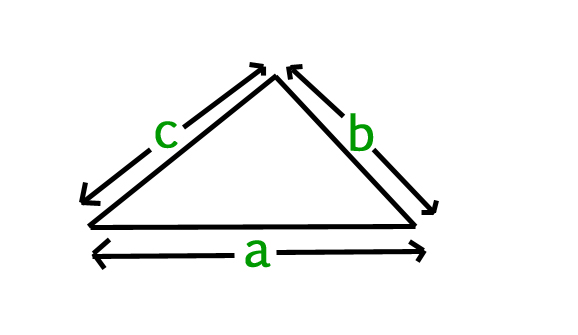GFG App
Open AppBrowser
Continue

# JavaScript program to find perimeter of a triangle

Given the side (a, b, c) of a triangle and we have to find the perimeter of a triangle.

Perimeter: Perimeter of a triangle is the sum of the length of the side of a triangle.Where a, b, and c are lengths of sides of a triangle. The perimeter of a triangle can be simply evaluated using the following formula:

`Perimeter = (a + b + c)`

Examples:

```Input : a = 2.0, b = 3.0, c = 5.0
Output : 10.0

Input : a = 5.0, b = 6.0, c = 7.0
Output : 18.0```

Example: Below is the implementation of above approach:

## Javascript

 ` `

Output:

```10.0
```

Time Complexity: O(1).
Auxiliary Space: O(1).

My Personal Notes arrow_drop_up